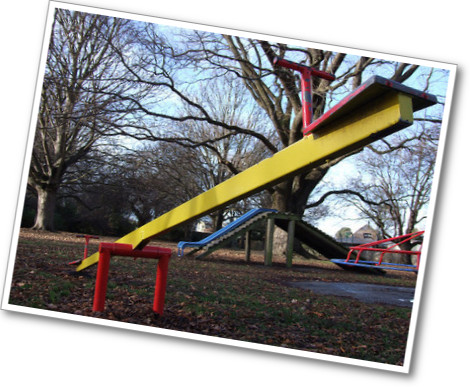# Gallery Problems Exercise

Student View (Opens in new window)# Gallery Overview

Allow students who have a clear understanding of the content thus far in the unit to work on Gallery problems of their choosing. You can then use this time to provide additional help to students who need review of the unit's concepts or to assist students who may have fallen behind on work.

# Gallery Descriptions

• Keep It Balanced
Students will use reasoning to complete some equations to make them true.
• Equation Sort
Students will sort equations into three groups: equations with one solution, equations with many solutions, and equations with no solutions
• On the Number Line
Students will use a number line to identify numbers that make an equation or inequality true.
• How Many Colors?
Students will write and solve an equation to find the number of different colored blocks in a box.
• Value of s
Students will use a property of equality to solve an equation with large numbers.
• Marbles in a Cup
Students are given information about the weight of a cup with two different amounts of marbles in it. They use this information to find the weight of the cup.
• When Is It True?
Students will use what they know about 0 and 1 to decide when a certain equation is true.